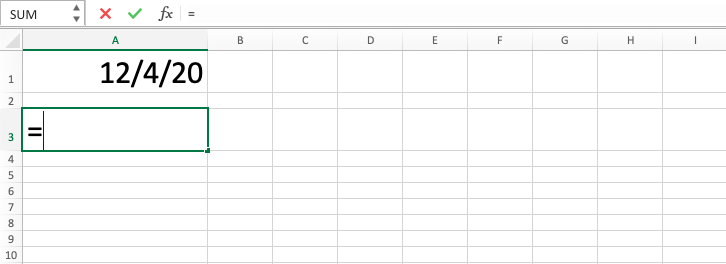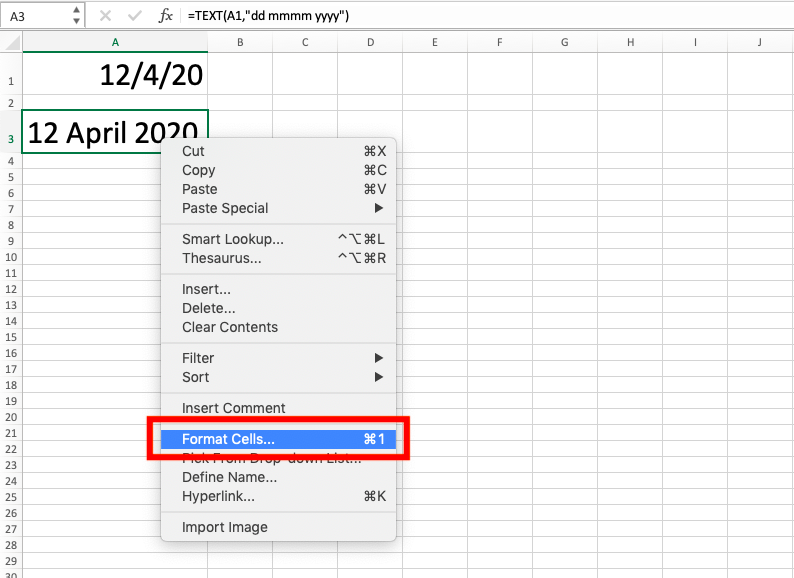TEXT Function in Excel - Compute Expert

# TEXT Function in Excel

In this tutorial, you will understand how to use TEXT function in excel. This formula is useful if you want to change the writing of a number to be a specific format in the form of a text.

## Why We Need to Learn About TEXT Function in Excel?

In doing writing to our number data, sometimes we want to change its format form so it can be as we want it for it to be more readable and also make it easier to be combined with our other text.

For example, when we want to do the writing of a number that represents monetary value in our text, then we often need to add the currency note on the data and change its format to become a specific money value digit form. This thing can be done if we change the format of the number to become a writing form of money value digit in general.

Another case example is, for instance, the desire to change the writing form of a date. Date numeral data has some forms of writing that can be found in different sequences of day, date, month, and year. If we need one of the date writing format and the date form available is not in line with the needs, then we need to do format changing process for the date digit data.

To make the process easier, there is TEXT function in excel that can be used to do numeral data format change process in this spreadsheet software. By understanding the function usage, then if you have many numeral data to be changed in its form, then this formula will help you do it in a significantly faster way.

## What is TEXT Function in Excel?

TEXT function in excel is a formula with usage objective to change digit data writing in this spreadsheet software with the format as needed. This formula is very useful if you often do numeral data processing in this spreadsheet software with various forms of writings.

Here is a little bit explanation for the inputs in this formula:

=TEXT(value, format_text)

Note:
• value = the number that the format wants to be changed
• format_text = the digit format that you desired

## How to Use TEXT Formula in Excel?

The following will explain how to write TEXT function in excel. This formula needs two main inputs which are number data that want to be changed on its writing and the writing format desired.

## Using TEXT Function in Excel

1. Type equal sign ( = ) in the cell where you want to put the result in2. Type TEXT (can be with large and small letters) and open bracket sign after =3. Type number or cell coordinate where the number which you want to process is in, after open bracket sign, then input comma sign ( , )4. Type your desired digit form between quotes sign ( “” )5. Type close bracket sign6. Press Enter
7. The process is done!## Exercise

After you have learned the steps from the tutorial, you can practice your understanding to be more adept by doing this exercise!

### Questions

Use TEXT formula to answer! Look at the Additional Note part if you are confused about the numeral data format input!
1. Change the content writing of cell B1 to have three decimal digits on B2!
2. Change the content writing of cell C1 to be day, month date year (e.g. Sunday, October 23 2012) on C2!
3. Change the content writing of cell D1 to percentage form on D2!

To see various digit forms that can be the reference for the usage of TEXT formula in this tutorial, there is the content of Format Cells menu that can be looked at.

To access the menu, right-click on a cell then choose Format Cells. Then choose Number Tab and click Custom. The access can also in Format option on the Home Tab.You can also directly do digit format editing in the menu if you want by choosing the appropriate form there and click OK.

Get updated excel info from Compute Expert by registering your email. It's free!